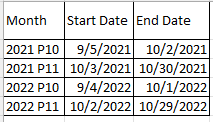cancel
Showing results for
Did you mean:Frequent Visitor

## Getting Same Period Last Year without date table

I have table with moving dates for months , below is the format. Now I need to calculate the time intelligence functions like Same Period Last Year, if the user selects 2022 P1,2022 P2, I need to display the values for same period last year like 2021 P1, 2021 P2. what is the easy way to do this?2 REPLIES 2Helper II

I really would recommend a date table, you could construct one from scratch and then add the periods required and it opens up many other options.  That also gives you the flexibility you get when your date ranges are non contiguous.

However...

You could use something like this:

``````MeasureName =
VAR PeriodStart = EDATE(SELECTEDVALUE('Date Table'[Start Date]), -12)
VAR PeriodEnd = EDATE(SELECTEDVALUE('Date Table'[End Date]), -12)

RETURN
SUMX(
VALUES('Date Table'[Month)),
CALCULATE(SUM('Fact Table'[Value]),
FILTER(VALUES('Fact Table'[Transaction Date]),
'Fact Table'[Transaction Date] >= PeriodStart &&
'Fact Table'[Transaction Date] <= PeriodEnd
)
)
)``````

I have not tested this code because I am at work and just throwing something together while data loaded, it could have some overlooked context issues so please test.  For averages you're better off making two measures like this and then dividing e.g. AvgPrice = DIVIDE([Total Price], [Total Units])Resident Rockstar

The easiest way to do this is with a date table- here is some additional information:

https://kteam.ch/why-almost-every-power-bi-report-needs-a-date-table/

https://learn.microsoft.com/en-us/dax/sameperiodlastyear-function-dax